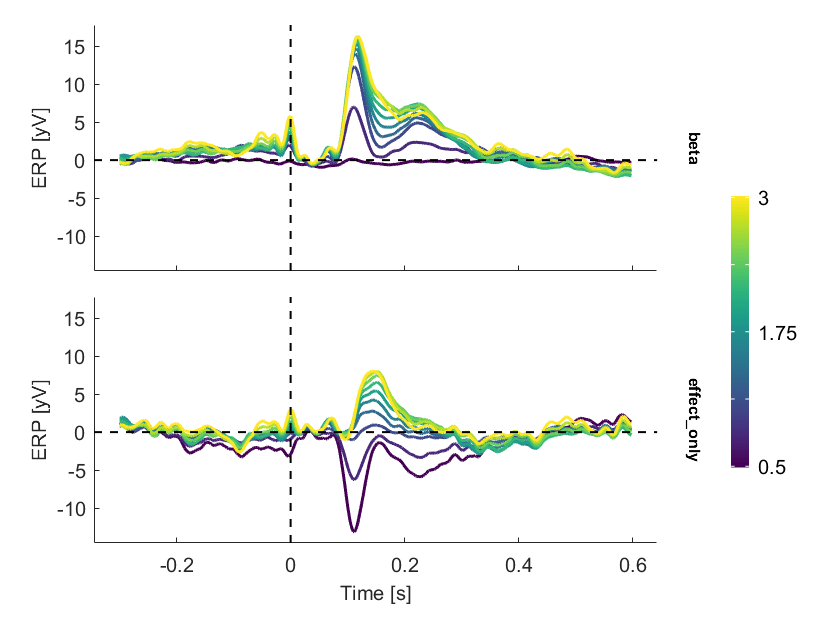init_unfold
Starting unfold toolbox. Adding subfolders and other toolboxes to path...
Warning: Adding folders named 'resources' to the path is not supported: C:\Users\behinger\cloud\PhD\deconvolution\lib\eeglab\functions\resources
eeglab: options file is C:\Users\behinger\eeg_options.m
EEGLAB: adding "ANTeepimport1.11 (Beta)" v1.10 (see >> help eegplugin_eepimport)
EEGLAB: adding "ANTeepimport" v1.13 (see >> help eegplugin_eepimport)
EEGLAB: adding "BDFimport" v1.10 (see >> help eegplugin_bdfimport) EEGLAB: adding "EYE-EEG" to the path; subfolders (if any) might be missing from the path
Warning: Name is nonexistent or not a directory: C:\Users\behinger\cloud\PhD\deconvolution\lib\eeglab\plugins\Fieldtrip-lite20191119\compat
Warning: Name is nonexistent or not a directory: C:\Users\behinger\cloud\PhD\deconvolution\lib\eeglab\plugins\Fieldtrip-lite20191119\forward
Warning: Name is nonexistent or not a directory: C:\Users\behinger\cloud\PhD\deconvolution\lib\eeglab\plugins\Fieldtrip-lite20191119\utilities
EEGLAB: adding "Fieldtrip-lite" to the path; subfolders (if any) might be missing from the path EEGLAB: adding "Fileio" to the path; subfolders (if any) might be missing from the path
EEGLAB: adding "bva-io" v1.5.13 (see >> help eegplugin_bva_io)
EEGLAB: adding "dipfit" v2.3 (see >> help eegplugin_dipfit)
EEGLAB: adding "eye-eeg-master" v0.85 (see >> help eegplugin_eye_eeg)
EEGLAB: adding "firfilt" v1.6.2 (see >> help eegplugin_firfilt)
EEGLAB: adding "firfilt" v2.1 (see >> help eegplugin_firfilt)
EEGLAB: adding "rERP" v? (see >> help eegplugin_rerp)
Warning: Adding folders named 'resources' to the path is not supported: C:\Users\behinger\cloud\PhD\deconvolution\lib\eeglab\functions\resources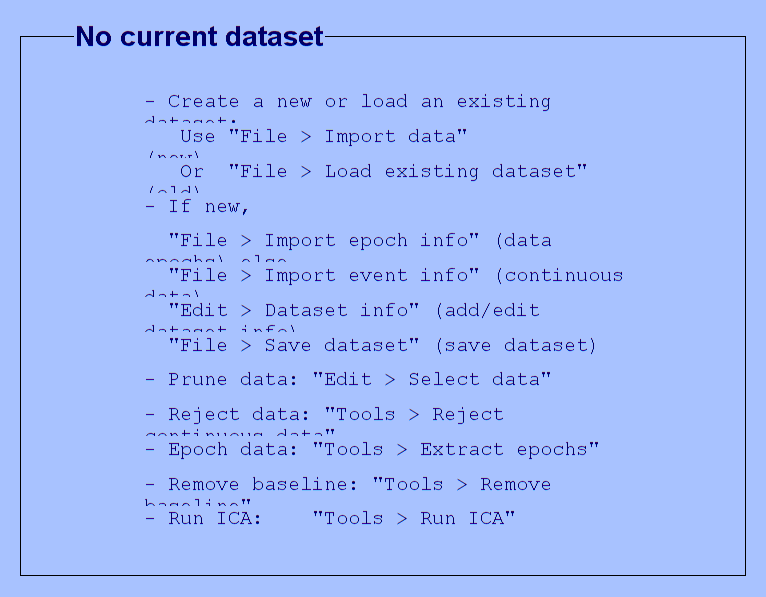Done.

# Introduction

Subjects are exploring a face stimulus. Using an eyetracker and the EEG-Eye toolbox we add markers of saccade onsets into our EEG-Data. The data were cleaned for eye-movement related artefacts using ICA.
In this tutorial we try to disentangle stimulus related ERPs and (micro)saccade related ERPs
Find the data at: https://osf.io/wbz7x/
EEG = struct with fields:
setname: 'face_saccades_opendata' filename: 'face_saccades_opendata_fig10.set' filepath: 'C:\Users\behinger\Downloads' subject: '' group: '' condition: '' session: [] comments: 'Data to re-create Figure 10 in Ehinger & Dimigen, 2018, BioRxiv. Please do not use this data for other purposes without asking first (olaf.dimigen@hu-berlin.de)' nbchan: 40 trials: 1 pnts: 2070402 srate: 500 xmin: 0 xmax: 4.1408e+03 times: [1×2070402 double] data: [40×2070402 single] icaact: [] icawinv: [] icasphere: [] icaweights: [] icachansind: [] chanlocs: [1×40 struct] urchanlocs: [] chaninfo: [1×1 struct] ref: 'averef' event: [1×7661 struct] urevent: [1×7661 struct] eventdescription: {'' '' '' '' '' '' '' '' '' '' '' '' '' ''} epoch: [] epochdescription: {} reject: [1×1 struct] stats: [1×1 struct] specdata: [] specicaact: [] splinefile: '' icasplinefile: '' dipfit: [] history: '↵EEG.setname='MSAFF_01';↵EEG = eeg_checkset( EEG );↵EEG = eeg_checkset( EEG );↵EEG.etc.eeglabvers = '14.1.2'; % this tracks which version of EEGLAB is being used, you may ignore it↵EEG = pop_loadset('filename','face_saccades_opendata.set','filepath','M:\\Dropbox\\shared folders_main\\deconv\\figures\\toolbox_paper\\redo_figure10_to_match_opendata\\');↵EEG = eeg_checkset( EEG );↵pop_eegplot( EEG, 1, 1, 1);↵EEG = pop_select( EEG,'nochannel',{'L-Dia-X-(px)' 'L-Dia-Y-(px)' 'R-Dia-X-(px)' 'R-Dia-Y-(px)'});↵EEG = eeg_checkset( EEG );↵EEG = pop_saveset( EEG, 'filename','face_saccades_opendata_fig10.set','filepath','M:\\Dropbox\\shared folders_main\\deconv\\figures\\toolbox_paper\\redo_figure10_to_match_opendata\\');↵EEG = eeg_checkset( EEG );↵EEG = pop_select( EEG,'nochannel',{'L-GAZE-X-(px)' 'L-GAZE-Y-(px)' 'R-GAZE-X-(px)' 'R-GAZE-Y-(px)'});↵EEG = eeg_checkset( EEG );↵EEG.etc.eeglabvers = '14.1.1'; % this tracks which version of EEGLAB is being used, you may ignore it' saved: 'justloaded' etc: [1×1 struct] datfile: []

# Defining the design

cfgDesign = [];
cfgDesign.eventtypes = {{'saccade'},{'stimonset'}}; % we model the saccadeonset and the stimulus
cfgDesign.formula = {'y ~ 1 + spl(sac_amplitude,10)','y~1'}; % 10 splines are generated; One needs to be careful to not overfit the data, regularization or cross validation can help here.
EEG = uf_designmat(EEG,cfgDesign);
Multiple events with separate model-formula detected Modeling 2871 event(s) of [saccade] using formula: y~1 The spline that got removed due to collinearity in the basis set (as intended) for the effect sac_amplitude has its peak at 0.969419 This does not mean that the event-intercept represents this value! Modeling 479 event(s) of [stimonset] using formula: y~1
The term spl(sac_amplitude,10) creates 10 splines over our predictor.
We can visualise the set of splines using:
spl = EEG.unfold.splines{1};
subplot(2,1,1),histogram(spl.paramValues,100),title('Histogram of saccadic amplitudes')
splineSet = spl.splinefunction(linspace(0,5,500),spl.knots);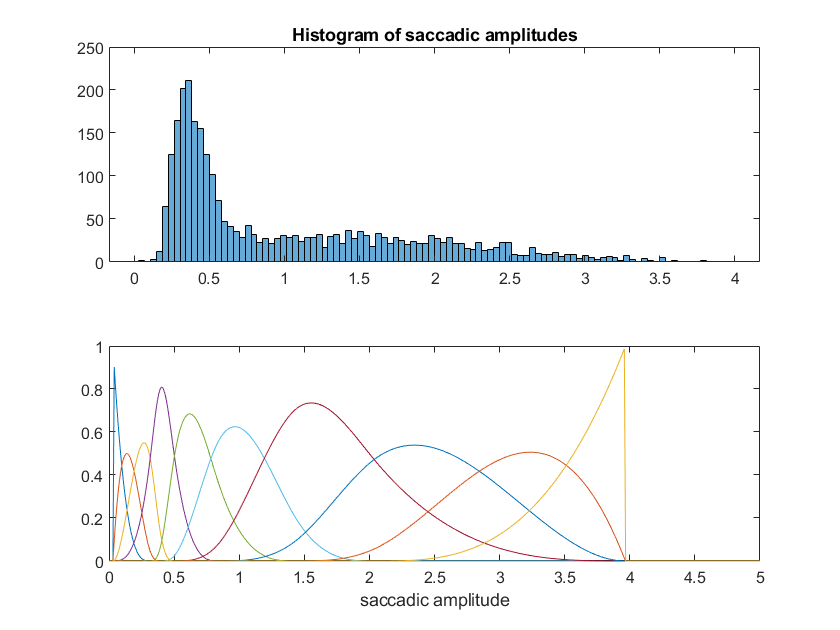The default setting is to put the splines at points of the quantiles of the variable. This ensures that in regions with a lot of data, lots of splines are generated and in regions with sparse data, broad splines are used. One could also put the splines in a linear or logarithmical way or define the knotsequence manually.
To understand better what basis function do, it might be helpful to have a look at the sorted designmatrix:
uf_plotDesignmat(EEG,'sort',1)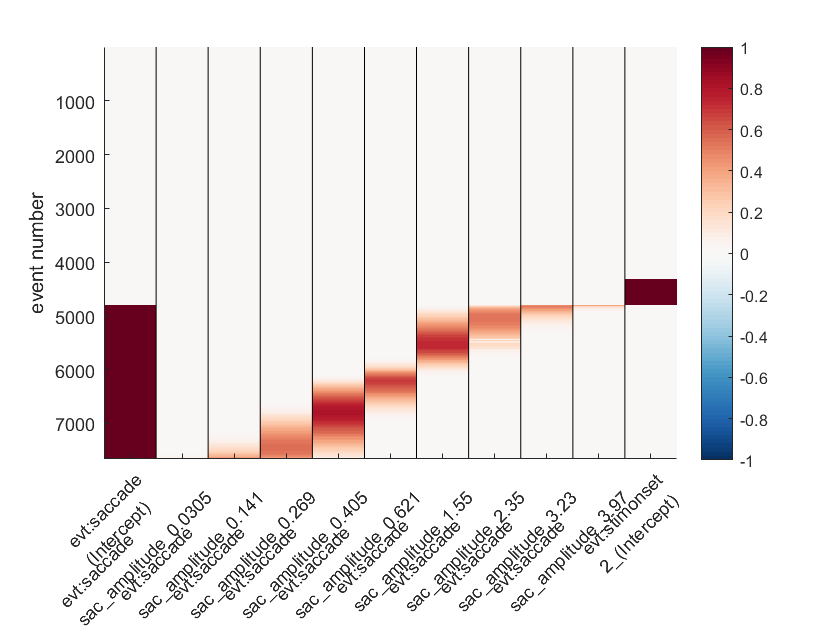The sorted designmatrix shows overlapping predictors, these are the splines for saccade amplitude. There is one predictor removed (between sac_amp 1.5 & 2.9). This is because we have an intercept in the model and else the model would be overcomplete. Not that the empty events (all grey rows) are events that are not modeled.
cfgTimeexpand = [];
cfgTimeexpand.timelimits = [-.3,.6];
EEG = uf_timeexpandDesignmat(EEG,cfgTimeexpand);
uf_timeexpandDesignmat(): Timeexpanding the designmatrix... ...done
winrej = uf_continuousArtifactDetect(EEG,'amplitudeThreshold',250);
Please wait. This might take several seconds... 164 segments were marked. A total of 552072 samples (26.66 percent of data) was marked as bad.
EEG = uf_continuousArtifactExclude(EEG,struct('winrej',winrej)); % we find data using a simple threshold tool. More complex algorithms or manual cleaning is recommended!
Portions of data removed split up by each event in EEG.event Type: S 99 modelled eventtime: 863.10s rejected eventtime: 97.69s percent removed: 11.3% Type: S100 modelled eventtime: 0.90s rejected eventtime: 0.90s percent removed: 100.0% Type: S200 modelled eventtime: 0.90s rejected eventtime: 0.00s percent removed: 0.0% Type: buttonpress modelled eventtime: 431.10s rejected eventtime: 63.68s percent removed: 14.8% Type: fixation modelled eventtime: 766.25s rejected eventtime: 113.69s percent removed: 14.8% Type: saccade modelled eventtime: 765.77s rejected eventtime: 113.73s percent removed: 14.9% Type: stimonset modelled eventtime: 431.10s rejected eventtime: 66.93s percent removed: 15.5% Removing 26.67% of rows from design matrix (filling them with zeros)
EEG= uf_glmfit(EEG,'channel',32);
uf_glmfit(): Fitting deconvolution model...solving the equation system solving electrode 32 (of 1 electrodes in total)... 77 iterations, took 1.8s LMfit finished

# Plot the results

Currently the spline-predictor is still separated in intercept + 9 spline-betas. The relationship for e.g. the P100 can be visualized. We start with a simple investigation of the raw betas, without undoing the spline-basisset
ufresult= uf_condense(EEG);
sac_amp_ix = cellfun(@(x)~isempty(x),(strfind({ufresult.param.name},'sac_amplitude'))); % get all betas related to sac_amplitude
timeix = get_min(0.115,ufresult.times); % somewhere around the p100
splinevalue = [ufresult.param(sac_amp_ix).value];
figure;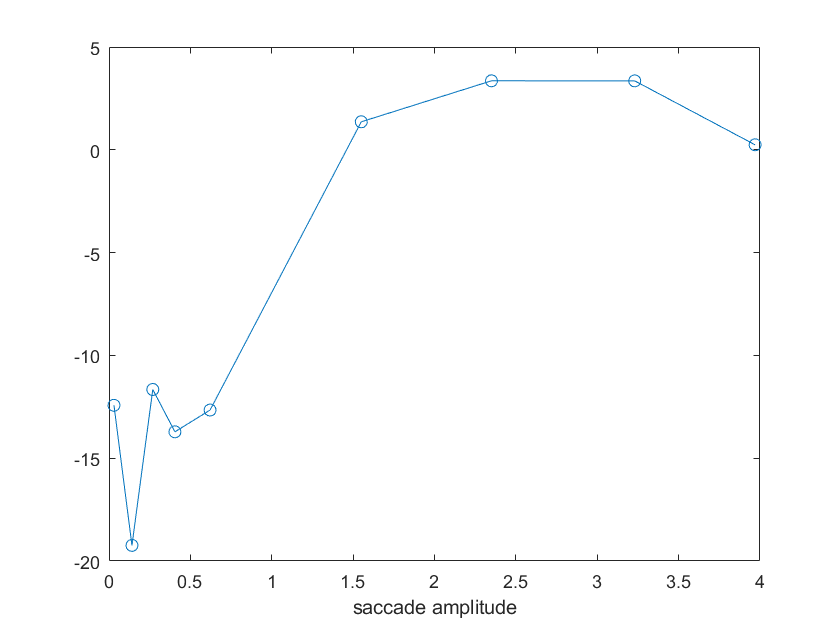This plot is still lacking the intercept (a DC-offset) and of course, these betas represent only weights to the above plotted set of basisfunctions.
In order to get a better picture, let's multiply the betas with the basis function to get an estimate in the predictor-value-domain.
ufresult= uf_predictContinuous(ufresult,'predictAt',{{'sac_amplitude',linspace(0.3,5,100)}});
sac_amp_ix = cellfun(@(x)~isempty(x),(strfind({ufresult.param.name},'sac_amplitude'))); % get all betas related to sac_amplitude
y = ufresult.beta(32,timeix,sac_amp_ix);
% we could add the intercept; it is a constant offset
%y = y + ufresult.beta(1,timeix,1);
plot([ufresult.param(sac_amp_ix).value],squeeze(y)),xlabel('saccade amplitude'),title(sprintf('Non-linear relationship between ERP and saccadic amplitude @ %.2f s',ufresult.times(timeix)))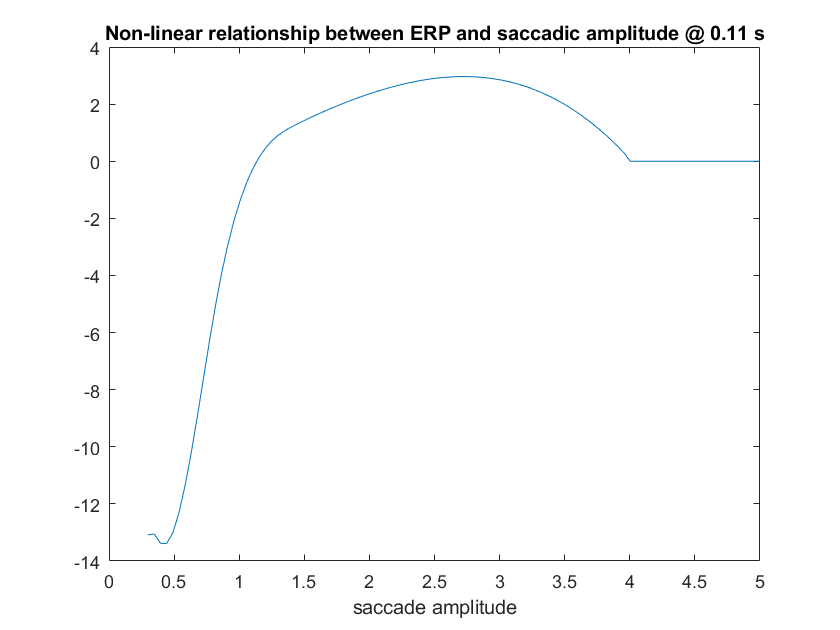It is clear from this plot, that there is a strongly non-linear relationship between saccade amplitude and ERP at around 100ms.
It's important to remember here, that above 3° saccade amplitude only very few saccades remain. It is therefore important to not overinterpret this downward trend!
To really drive home the point we add the weighted splines to the plot:
ufresult= uf_condense(EEG);
sacX =linspace(0.3,5,500); % at which points to evaluate?
splineSet = spl.splinefunction(sacX,spl.knots);
% remove the spline that was moved into the intercept
splineSet(:,ufresult.unfold.splines{1}.removedSplineIdx) = [];
beta = squeeze(ufresult.beta(32,timeix,2:end-1)); % 2: to remove the intercept, end-1 because of the stimulus event predictor
splineEvaluated = bsxfun(@times,splineSet,beta'); % weight the basis functions by the betas, but don't add them
plot(sacX,splineEvaluated); hold all;
plot(sacX,sum(splineEvaluated,2),'k','LineWidth',1.5), % add the basis functions at each saccade-amplitude to get the modelfit
xlabel('saccade amplitude'),title(sprintf('Non-linear relationship between ERP and saccadic amplitude @ %.2f s',ufresult.times(timeix)))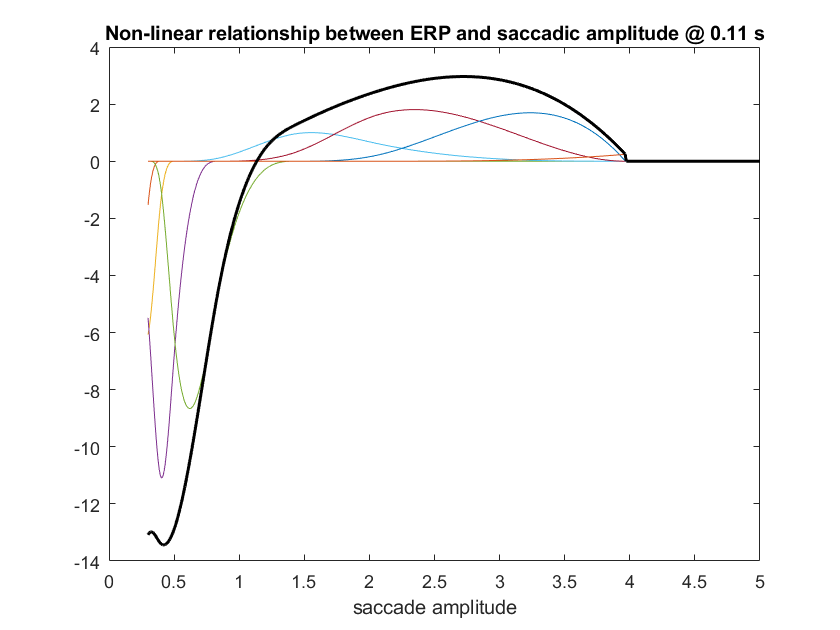As one can also see, the modelfit is a bit rough at the edges. this is to be expected because there is not much data for very small and very large splines.
% The default parameters plot the predictor at its quantiles
ufpredict = uf_predictContinuous(ufresult); % evaluate the splines at the quantiles
contValueSelect = 1×10
0.2812 0.3358 0.3846 0.4486 0.5292 0.7558 1.1117 1.4832 1.8599 2.3160
plotting all parameters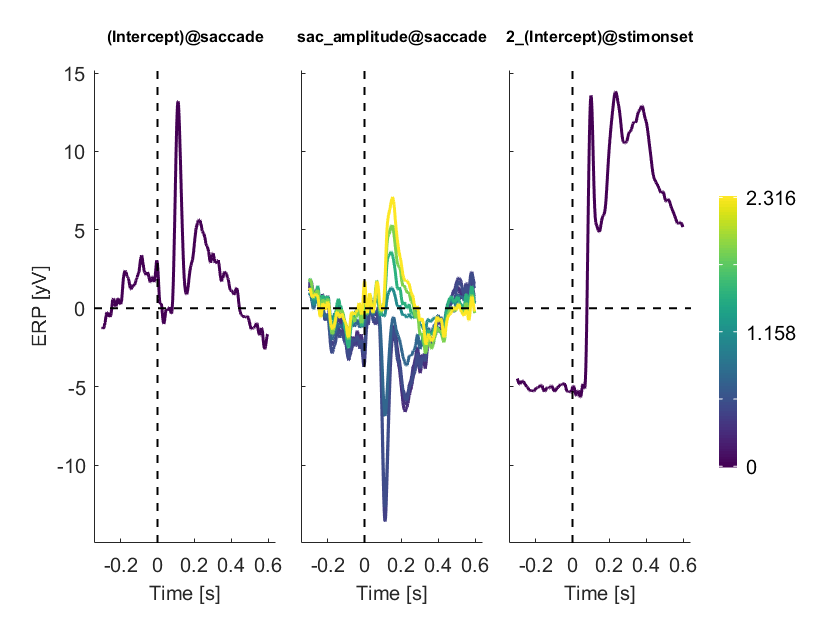Important notes to this plot:
• The lines represent saccade-amplitudes at the quantiles of the saccade amplitude distribution.
• There are outliers/bad estimates at the extremes. The yellow-curve has a very high noise level. This is because the last spline is only informed by a small amount of trials, it is usually highly unstable.
In order to get a more representative picture, we can utilize the 'predictAt' option in the plot and put a linear spacing excluding the extremata
ufpredict= uf_predictContinuous(ufresult,'predictAt',{{'sac_amplitude',linspace(0.5,3,10)}});